Technical Article

# Introduction to Clock Domain Crossing: Double Flopping

October 05, 2018 by Dr. Steve Arar

## This article will discuss a well-known technique called “double flopping” to transfer a single-bit control signal between two clock domains.

This article will discuss a well-known technique called “double flopping” to transfer a single-bit control signal between two clock domains.

It is common to employ several clock signals in a digital system. Since the clock signals of different clock domains are independent in general, transferring data between the different clock domains can be a challenging task. This article will discuss a well-known technique called “double flopping” to transfer a single-bit control signal between two clock domains.

### Why Do We Need Multiple Clocks?

The general digital design methodology recommends using one clock signal for the entire system mainly because it simplifies both the design procedure and the system timing analysis. However, this methodology doesn’t always give the most efficient solution and sometimes it’s not even possible to have a single clock for the whole system. For example, consider an FPGA design operating at 20 MHz which is communicating with two external devices using interfaces operating at 100 MHz and 150 MHz. Here, we have to deal with three different clock frequencies. Note that the clock frequency of the interfaces can be predefined and we may not be able to choose it based on the clock utilized inside the FPGA.

Sometimes, we may be able to choose the clock frequency for the different parts of the system but, even in this case, it may not be a good idea to operate the entire system at a given clock frequency. For example, assume that the whole system can be operated at 20 MHz except for a subsystem which needs a 100-MHz clock. If we decide to use one clock signal for the entire system, then we would have to operate the system at 100 MHz to accommodate the highest clock rate available in the system. Obviously, this is not reasonable because not only we’ve overdesigned a large portion of the system (parts that could be operated at 20 MHz) but also we’ve unnecessarily increased the system dynamic power consumption. As you can see, there are many circumstances in which we need to employ different clock rates for different parts of the system.

A section of the design in which all the synchronous elements, such as flip-flops and RAMs, use the same clock signal is referred to as a clock domain. Having different clock domains can be beneficial but is not as easy as it seems to be. The next section discusses some of the problems that we may face when using a multiple-clock system.

### The Metastability Problem

Assume that we have two sections of logic, A and B, that operate at 50 MHz and 100 MHz, respectively. This is shown in Figure 1.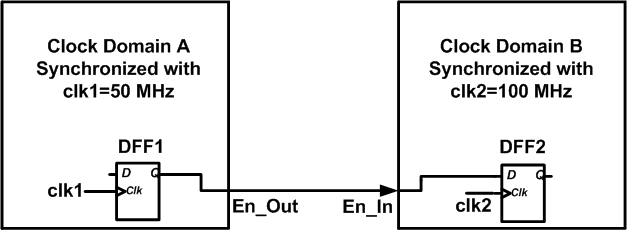##### Figure 1

In our simple example, the B section has an input, En_In, which is connected to the En_Out output of the A section. This connection corresponds to an active-high enable signal that initiates an algorithm in B after a particular operation is done by A. Figure 1 shows the register generating the enable signal in A and the register receiving it in B.

Assume that the clock waveforms are as shown in Figure 2 and the system is rising edge-triggered. Since the En_Out signal is generated by the A clock domain, its low-to-high transition can occur after a rising edge of clk1 as shown in the figure. The delay between the clk1 rising edge and the En_Out transition corresponds to the clock-to-Q delay ($$T_{clk-to-Q, DFF1}$$) of the flip-flop in the A logic section. Now, we expect that the DFF2 register in the B domain will sample the enable signal at the next rising edge of clk2 at $$t=t_2$$. The sampling will successfully happen provided the timing requirements of the DFF2 are met, i.e. $$t_1+T_{clk-to-Q, \; DFF1} \leq t_2-T_{setup, \; DFF2}$$.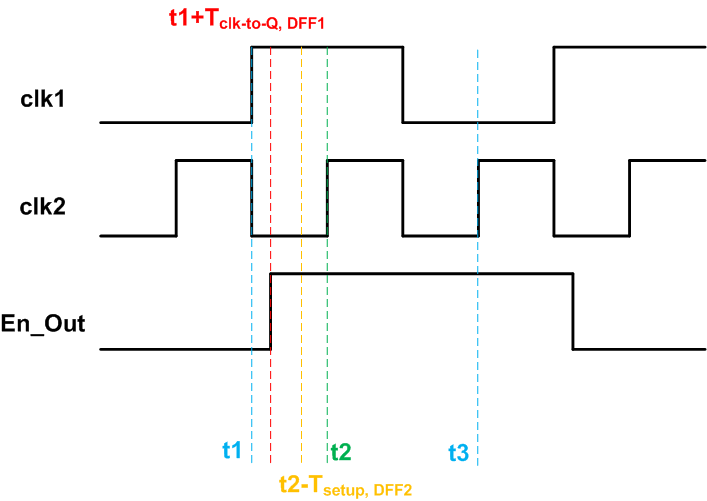##### Figure 2

In Figure 2, the condition $$t_1+T_{clk-to-Q, \; DFF1} \leq t_2-T_{setup, \; DFF2}$$ is satisfied but this is not always the case. Note that the clock signals of different clock domains are independent in general. We don’t know their phase relationship and the waveforms can be as shown in Figure 3. In this case, the low-to-high transition of the enable signal is so close to the rising edge of clk2 that the condition $$t_1+T_{clk-to-Q, \; DFF1} \leq t_2-T_{setup, \; DFF2}$$ is not satisfied.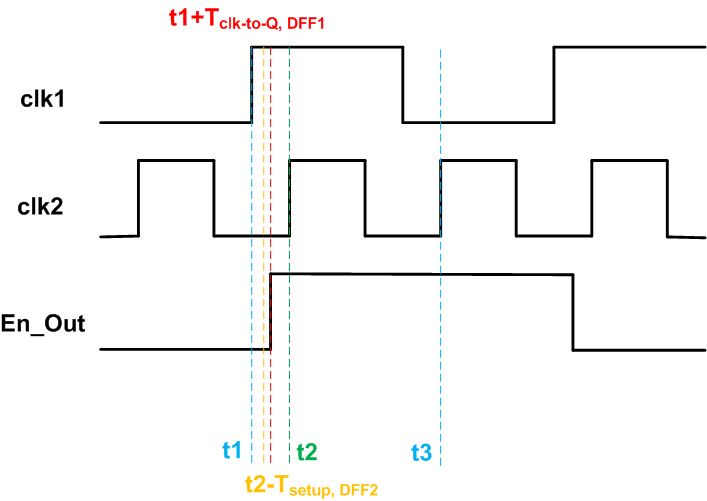##### Figure 3

Since the input data of DFF2 has changed within the setup time, the register behavior will be unpredictable. Due to the setup time violation, the register output voltage could be the value representing a logic high, a logic low, or even worse a value between the logic high and logic low voltages. These three cases are possible while the input data was actually logic high at the corresponding clock edge. Similarly, the output value of the register will be unpredictable, when the register hold time is violated, i.e. En_Out changes within a time window after the active clock edge defined by the register hold time. When the output of the register becomes suspended at a voltage between the logic high and logic low voltages, we say that the flip-flop has entered a metastable state.

Let’s examine the three possible cases from timing violation of Figure 3 individually:

• As the first case, assume that the output value of the DFF2 goes to logic high with the clk2 rising edge at $$t=t_2$$. In this case, there is no error and the flip-flop contains valid data although we had setup time violation. The data transitions as expected with no error.
• The second case: assume that the DFF2 output goes to logic low with the clk2 rising edge at $$t=t_2$$. In this case, the enable signal is not successfully sampled in the B clock domain. However, this won’t be a problem because En_Out comes from the A clock domain and it will be high for at least one period of clk1 as shown in Figure 3. Therefore, the next rising edge of clk2 at $$t=t_3$$ will sample the En_Out value correctly. For this clock edge, the timing requirements of DFF2 will be satisfied because En_Out has not changed for more than one period of clk2. In this case, we are sampling En_Out about one clock period later than it actually transitioned. However, this is not a problem because the clocks of the two clock domains were assumed to be independent and we didn’t make any assumption about the arrival time of the En_Out signal. In fact, the circuit in the B clock domain realizes the end of calculations of the A clock domain with an extra delay of one clk2 period.
• Next assume that the DFF2 register enters the metastable state. In this case, the register output becomes suspended at a voltage between the logic high and logic low voltages but this will be temporary. The flip-flop will eventually exit the metastable state and go to logic high or logic low. The time required to exit the metastable state is known as the resolution time $$T_r$$. This is shown in Figure 4. In this figure, the setup time violation has occurred and the flip flop has entered the metastable state for a time interval of $$T_r$$. After $$T_r$$, the flip flop output will go to either logic high ($$Q2_{meta-to-1}$$) or logic low ($$Q2_{meta-to-0}$$).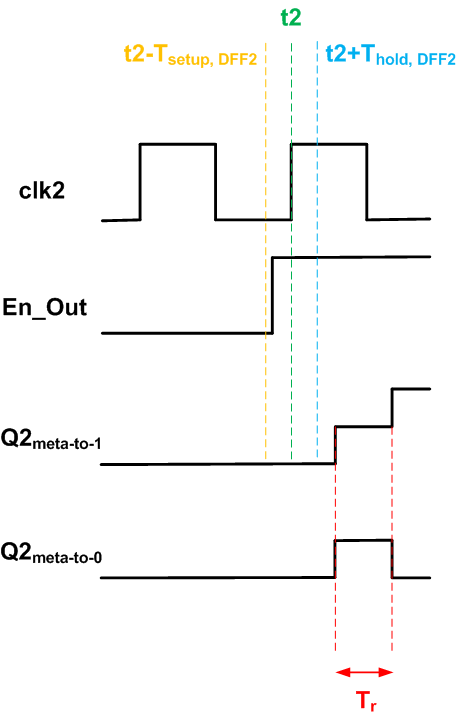##### Figure 4

The resolution time is not deterministic and is described as a probability distribution function

$$P(T_r)=e^{\frac{-T_r}{\tau}}$$

Where $$\tau$$ is the “decay time constant” and is determined by the electrical characteristics of the flip flop. A typical value for this parameter will be about a fraction of one nanosecond.

The above equation gives the probability of remaining in the metastable state for a time interval equal to $$T_r$$ after the sampling clock edge. Due to the exponential characteristic of the equation, the probability will rapidly decrease as we increase the value of $$T_r$$. For example, for $$\tau =0.5$$ ns and $$T_r = 5$$ ns, we obtain a probability of $$\approx 4.5 \times 10^{-5}$$.

To summarize, we cannot prevent metastability from happening because the clock signals of the two clock domains are independent from each other. However, if we provide the flip-flop with sufficiently large resolution time, it will resolve to a stable state with a high probability. Hence, if our design includes flip-flops that could enter the metastability state, we should give the flip-flop enough time to exit the metastability. Then, we can safely propagate the value of the flip-flop to the downstream logic cells. Note that using a metastable value can lead the entire system into an unknown state. It can lead to a high current flow and even chip burnout in the worst case. Hence, we should avoid feeding unstable data to the system.

### Double Flopping

We saw that giving the flip-flop a sufficient time can greatly reduce the chance of remaining in the metastable state. Let’s see how this can be used to avoid propagating metastable data in the system. Consider the block diagram in Figure 5. This shows a typical path in the B clock domain of Figure 1 that receives and processes the En_In signal.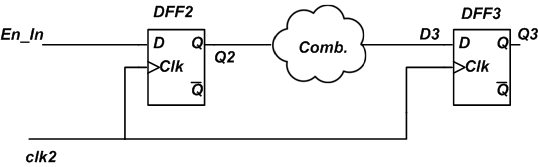##### Figure 5

The minimum clock period that can be used to operate this circuit will be

$$T_{clk, \; min} = T_{clk-to-Q} + T_{comb, \; max} + T_{setup}$$

where $$T_{clk-to-Q}$$ and $$T_{setup}$$ are the clock-to-Q and the setup time of the flip-flops and $$T_{comb, \; max}$$ is the maximum delay that the combinational circuit, “Comb.”, exhibits. This equation is obtained by assuming that the output data of DFF2 is stable. If it is not, we have to consider some resolution time as in the following equation:

$$T_{clk, \; min} = T_{clk-to-Q} + T_r + T_{comb, \; max} + T_{setup}$$

The value of the resolution time will determine the probability of coming out of the metastable state. Assume that the period of clk2 is $$T_{clk2}$$. Then, the value of the available resolution time will be

$$T_r = T_{clk2} - \big ( T_{clk-to-Q} + T_{comb, \; max} + T_{setup} \big )$$

To reduce the probability of remaining in a metastable state, we should increase $$T_r$$. With a given clock period $$T_{clk2}$$, the only design option will be minimizing the parameter $$T_{comb, \; max}$$. Therefore, we’d better put the “Comb.” block after the DFF3 flip-flop as shown in Figure 6. In this way, $$T_{comb, \; max}$$ will be theoretically zero for the path between DFF2 and DFF3. Hence, we’ll have the maximum possible resolution time.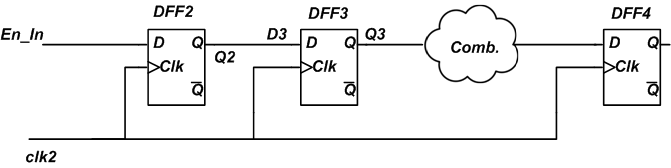##### Figure 6

This technique is called double flopping and is widely used when transferring control signals like the above enable signal between two clock domains. Note that the extra register will introduce another delay of one clock period to the enable signal captured by the B clock domain. However, this delay is worth the benefit of avoiding metastable states in the system.

This article discussed passing an enable signal from a slow clock domain to a fast clock domain. You may need to get familiar with several other techniques such as passing a control signal from fast to the slow clock domain, the hand shaking technique and FIFO-based data transfer between the clock domains. You can find some details in Chapter 16 of RTL Hardware Design Using VHDL: Coding for Efficiency, Portability, and Scalability and Chapter 6 of Advanced FPGA Design: Architecture, Implementation, and Optimization.

### Summary

• There are many circumstances in which we need to employ different clock rates for different parts of the system.
• Since the clock signals of different clock domains are independent in general, transferring data between the different clock domains can be a challenging task.
• The output value of a register will be unpredictable when a setup time or hold time violation occurs. It could hold the value representing a logic high, a logic low, or even worse a value between the logic high and logic low voltages.
• The time required to exit the metastable state is known as the resolution time $$T_r$$.
• If our design includes flip-flops that could enter the metastability state, we should give the flip-flop enough time to exit the metastability.
• The “double flopping” technique is widely used to transfer single-bit control signals between two clock domains.